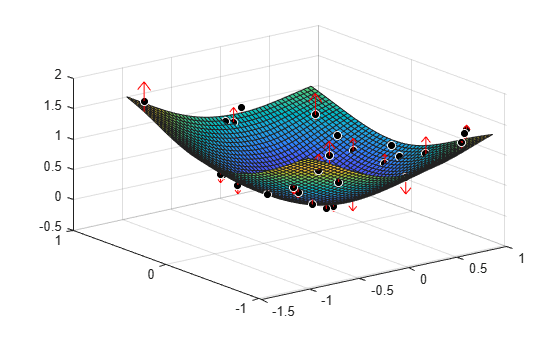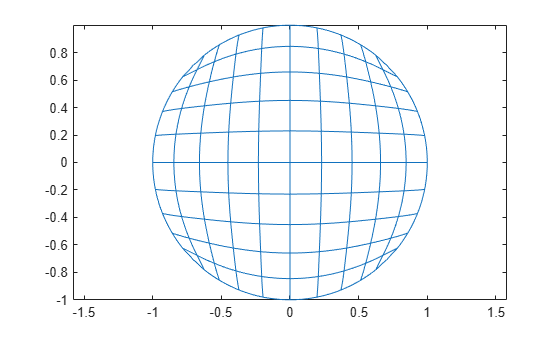# tpaps

Thin-plate smoothing spline

## Syntax

``st = tpaps(x,y)``
``st = tpaps(x,y,p)``
``[...,P] = tpaps(...) ``

## Description

example

````st = tpaps(x,y)` is the stform of a thin-plate smoothing spline f for the given data sites `x(:,j)` and the given data values `y(:,j)`. The `x(:,j)` must be distinct points in the plane, the values can be scalars, vectors, matrices, even ND-arrays, and there must be exactly as many values as there are sites.The thin-plate smoothing spline f is the unique minimizer of the weighted sum$pE\left(f\right)+\left(1-p\right)R\left(f\right)$with E(f) the error measure$E\left(f\right)=\sum _{j}{|y\left(:,j\right)-f\left(x\left(:,j\right)\right)|}^{2}$and R(f) the roughness measure$R\left(f\right)={\int \left(|{D}_{1}{D}_{1}f|}^{2}+2{|{D}_{1}{D}_{2}f|}^{2}+{|{D}_{2}{D}_{2}f|}^{2}\right)$Here, the integral is taken over all of R2, |z|2 denotes the sum of squares of all the entries of z, and Dif denotes the partial derivative of f with respect to its i-th argument, hence the integrand involves second partial derivatives of f. The function chooses the smoothing parameter `p` so that `(1-p)/p` equals the average of the diagonal entries of the matrix `A`, with ```A + (1-p)/p*eye(n)``` the coefficient matrix of the linear system for the `n` coefficients of the smoothing spline to be determined. This ensures staying in between the two extremes of interpolation (when `p` is close to `1` and the coefficient matrix is essentially `A`) and complete smoothing (when `p` is close to `0` and the coefficient matrix is essentially a multiple of the identity matrix). This serves as a good first guess for `p`.```

example

````st = tpaps(x,y,p)` also inputs the smoothing parameter, `p`, a number between 0 and 1. As the smoothing parameter varies from 0 to 1, the smoothing spline varies, from the least-squares approximation to the data by a linear polynomial when `p` is `0`, to the thin-plate spline interpolant to the data when `p` is `1`.```
````[...,P] = tpaps(...) ` also returns the value of the smoothing parameter used in the final spline result whether or not you specify `p`. This syntax is useful for experimentation in which you can start with ```[pp,P] = tpaps(x,y)``` and obtain a reasonable first guess for `p`.```

## Examples

collapse all

The following code obtains values of a smooth function at 31 randomly chosen sites, adds some random noise to these values, and then uses `tpaps` to recover the underlying exact smooth values. To illustrate how well `tpaps` does in this case, the code plots, in addition to the smoothing spline, the exact values (as black balls) as well as each arrow leading from a smoothed value to the corresponding noisy value.

```rng(23); nxy = 31; xy = 2*(rand(2,nxy)-.5); vals = sum(xy.^2); noisyvals = vals + (rand(size(vals))-.5)/5; st = tpaps(xy,noisyvals); fnplt(st), hold on avals = fnval(st,xy); plot3(xy(1,:),xy(2,:),vals,'wo','markerfacecolor','k') quiver3(xy(1,:),xy(2,:),avals,zeros(1,nxy),zeros(1,nxy), ... noisyvals-avals,'r'), hold off```The following code uses an interpolating thin-plate spline to vector-valued data values to construct a map, from the plane to the plane, that carries the unit square $\left\{\mathit{x}:|\mathit{x}\left(\mathit{j}\right)|\le 1,\mathit{j}=\mathrm{1}:2\right\}$ approximately onto the unit disk $\left\{\mathit{x}:{\mathit{x}\left(1\right)}^{2}+{\mathit{x}\left(2\right)}^{2}\le 1\right\}$.

```n = 64; t = linspace(0,2*pi,n+1); t(end) = []; values = [cos(t); sin(t)]; centers = values./repmat(max(abs(values)),2,1); st = tpaps(centers, values, 1); fnplt(st), axis equal```Note the choice of `1` for the smoothing parameter here, to obtain interpolation.

## Input Arguments

collapse all

Data sites of data values `y` to be fit, specified as a vector or as a cell array for multivariate data. Spline f is created with knots at each data site `x` such that f(`x(j)`) = `y(:,j)` for all values of `j`.

For multivariate, gridded data, you can specify `x` as a cell array that specifies the data site in each variable dimension: f(`x1(i),x2(j),...xn(k)`) = `y(:,i,j,...,k)`.

Data values to fit during creation of the spline, specified as a vector, matrix, or array. Data values `y(:,j)` can be scalars, matrices, or n-dimensional arrays. Data values given at the same data site `x` are averaged.

Data Types: `single` | `double`

Smoothing parameter, specified as a scalar value between 0 and 1 or as a cell array of values for multivariate data. You can also specify values for the roughness measure weights λ by providing `p` as a vector. To provide roughness measure weights for multivariate data, use a cell array of vectors. If you provide an empty array, the function chooses a default value for `p` based on the data sites `x` and the default value of `1` for the roughness measure weight λ.

The smoothing parameter determines the relative weight to place on the contradictory demands of having f be smooth or having f be close to the data. For `p` = 0, f is the least-squares straight-line fit to the data. For `p` = 1, f is the variational, or natural, cubic spline interpolant. As `p` moves from 0 to 1, the smoothing spline changes from one extreme to the other.

The favorable range for `p` is often near 1/(1 + h3/6), where h is the average spacing of the data sites. The function chooses a default value for `p` within this range. For uniformly spaced data, you can expect a close fit with p = 1(1 + h3/60) and some satisfactory smoothing with p = 1/(1 + h3/0.6). You can input `p > 1`, but this choice leads to a smoothing spline even rougher than the variational cubic spline interpolant.

If the input `p` is negative or empty, then the function uses the default value for `p`.

You can specify the roughness measure weights λ alongside the smoothing parameter by providing `p` as a vector. This vector must be the same size as `x`, with the `i`th entry the value of λ on the interval `(x(i-1)...x(i))`, for ```i = 2:length(x)```. The first entry of the input vector `p` is the desired value of the smoothness parameter `p`. By providing roughness measure weights, you can make the resulting smoothing spline smoother (with larger weight values) or closer to the data (with smaller weight values) in different parts of the interval. Roughness measure weights must be nonnegative.

If you have difficulty choosing `p` but have some feeling for the size of the noise in `y`, consider using `spaps(x,y,tol)` instead. This function chooses `p` such that the roughness measure is as small as possible, subject to the condition that the error measure does not exceed `tol`. In this case, the error measure usually equals the specified value for `tol`.

Data Types: `single` | `double`

## Output Arguments

collapse all

Spline, returned as a structure with these fields.

Form of the spline, returned as `st-tp00`, `st-tp10`, `st-tp01`, or `st-tp`.

Sequence of sites, returned as a matrix or as an array for multivariate data.

Coefficients of polynomials for each piece, returned as a matrix or as an array for multivariate data.

Number of sequence of sites.

Number of polynomial pieces describing the spline, returned as a scalar or as a vector of numbers of pieces in each variable for multivariate data.

Dimensionality of the target function, returned as a scalar.

Basic interval for the stform that contains all the given centers, returned as an array.

Smoothing parameter used to calculate the spline, returned as a scalar or as a cell array of scalar values for multivariate data. `P` is between `0` and `1`.

## Limitations

The determination of the smoothing spline involves the solution of a linear system with as many unknowns as there are data points. Since the matrix of this linear system is full, the solving can take a long time even if, as is the case here, an iterative scheme is used when there are more than 728 data points. The convergence speed of that iteration is strongly influenced by `p`, and is slower the larger `p` is. So, for large problems, use interpolation, i.e., `p` equal to 1, only if you can afford the time.

## Version History

Introduced in R2006b